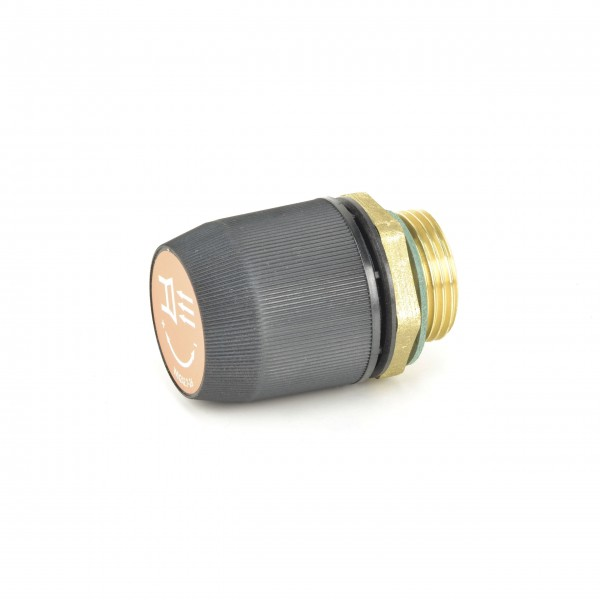# 73600099613 Vacuum Regulating ValvesDelivery time: 1 - 3 business days

• 73600099613
Becker Original Vacuum Regulating Valve 73600099613 Pressure & vacuum valves regulate... more
Product information "73600099613 Vacuum Regulating Valves"

Becker Original Vacuum Regulating Valve 73600099613
Pressure & vacuum valves regulate the operating pressure within a determined range. This valve is used within the pressure / vacuum range of 0 ... -0.6 bar. The connection size is: 1".

 Connection size 1: 1" ST-number: ST613-0-1 Type of valve: Vacuum Regulating Valve Pressure-/ Vacuumrange [bar]: 0 ... -0.6
 DVT 3.100 Order number: DVT3.100 DVT 3.100 (Index A) Order number: DVT3.100-A DVT 3.100 (Index B) Order number: DVT3.100-B DVT 3.100 (Index C) Order number: DVT3.100-C DVT 3.100 (Index D) Order number: DVT3.100-D DVT 3.100 (Index W) Order number: DVT3.100-W DVT 3.140 Order number: DVT3.140 DVT 3.140 (Index A) Order number: DVT3.140-A DVT 3.140 (Index B) Order number: DVT3.140-B DVT 3.140 (Index C) Order number: DVT3.140-C DVT 3.140 (Index D) Order number: DVT3.140-D DVT 3.140 (Index W) Order number: DVT3.140-W DVT 3.140/0-56 (Index D) Order number: DVT3.1400-56-D DVT 3.140/0-56 (Index W) Order number: DVT3.1400-56-WDVT 3.60 Order number: DVT3.60DVT 3.60 (Index A) Order number: DVT3.60-ADVT 3.60 (Index B) Order number: DVT3.60-BDVT 3.60 (Index C) Order number: DVT3.60-C DVT 3.60 (Index D) Order number: DVT3.60-DDVT 3.60 (Index E) Order number: DVT3.60-EDVT 3.60 (Index V) Order number: DVT3.60-V DVT 3.60 (Index W) Order number: DVT3.60-WDVT 3.80 Order number: DVT3.80DVT 3.80 (Index A) Order number: DVT3.80-ADVT 3.80 (Index B) Order number: DVT3.80-B DVX 3.60 Order number: DVX3.60DVX 3.60 (Index F) Order number: DVX3.60-FEP40/P40i (Index A) Order number: EP40P40i-AEPV40/P40i (Index A) Order number: EPV40P40i-A EPV40/PV40i (Index A) Order number: EPV40PV40i-AEV40/P40i (Index A) Order number: EV40P40i-AEV40/PV40i (Index A) Order number: EV40PV40i-AEV40/V40i (Index A) Order number: EV40V40i-AT 3.16 DS (Index G) Order number: T3.16DS-GT 3.16 DS (Index H) Order number: T3.16DS-HT 3.16 DSK (Index F) Order number: T3.16DSK-FT 3.16 DSK (Index G) Order number: T3.16DSK-GT 3.16 DSK (Index H) Order number: T3.16DSK-HT 3.25 DSK (Index F) Order number: T3.25DSK-FT 3.25 DSK (Index G) Order number: T3.25DSK-GT 3.25 DSK, CSA (Index F) Order number: T3.25DSKCSA-FT 3.25 DSK, CSA (Index G) Order number: T3.25DSKCSA-GT 3.40 DSK (Index F) Order number: T3.40DSK-FT 3.40 DSK (Index G) Order number: T3.40DSK-G T 4.10 DV Order number: T4.10DV T 4.16 DV Order number: T4.16DV T 4.25 DSK (Index A) Order number: T4.25DSK-A T 4.25 DSK (Index C) Order number: T4.25DSK-C T 4.25 DSK (Index X) Order number: T4.25DSK-X T 4.25 DSK (Index Z) Order number: T4.25DSK-Z T 4.25 DV (Index A) Order number: T4.25DV-A T 4.25 DV (Index C) Order number: T4.25DV-C T 4.25 DV (Index X) Order number: T4.25DV-X T 4.25 DV (Index Z) Order number: T4.25DV-Z T 4.40 DSK (Index A) Order number: T4.40DSK-A T 4.40 DSK (Index C) Order number: T4.40DSK-C T 4.40 DSK (Index X) Order number: T4.40DSK-X T 4.40 DSK (Index Z) Order number: T4.40DSK-ZT 4.40 DSK, /-34 (Index A) Order number: T4.40DSK-34-AT 4.40 DSK, /-34 (Index C) Order number: T4.40DSK-34-CT 4.40 DSK, /-34 (Index X) Order number: T4.40DSK-34-XT 4.40 DSK, /-34 (Index Z) Order number: T4.40DSK-34-ZT 4.40 DV (Index A) Order number: T4.40DV-A T 4.40 DV (Index C) Order number: T4.40DV-C T 4.40 DV (Index X) Order number: T4.40DV-X T 4.40 DV (Index Z) Order number: T4.40DV-Z VT 4.10 Order number: VT4.10 VT 4.16 Order number: VT4.16 VT 4.16/0-34 Order number: VT4.160-34 X 4.10 DV Order number: X4.10DV X 4.16 DV Order number: X4.16DV X 4.25 DSK Order number: X4.25DSKX 4.25 DSK (Index C) Order number: X4.25DSK-C X 4.40 DSK Order number: X4.40DSKX 4.40 DSK (Index C) Order number: X4.40DSK-C
Viewed